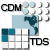## Dataset: Aggregated 7 day CBOFS Fields Forecast/Best Time Series

• Data format: netCDF
• Data type: GRID
• Naming Authority: gov.noaa.nos.co-ops
• ID: CBOFS/fmrc/Aggregated_7_day_CBOFS_Fields_Forecast_best.ncd

### Variables:

• Vocabulary [CF-1.0]:
• Pair (millibar) = surface air pressure = surface_air_pressure
• Uwind (meter second-1) = surface u-wind component = grid_eastward_wind
• Vwind (meter second-1) = surface v-wind component = grid_northward_wind
• angle (radians) = angle between XI-axis and EAST =
• f (second-1) = Coriolis parameter at RHO-points =
• h (meter) = bathymetry at RHO-points = sea_floor_depth
• oxygen (millimole_oxygen meter-3) = dissolved oxygen concentration =
• pm (meter-1) = curvilinear coordinate metric in XI =
• pn (meter-1) = curvilinear coordinate metric in ETA =
• salt = salinity = sea_water_salinity
• temp (Celsius) = potential temperature = sea_water_temperature
• u (meter second-1) = u-momentum component = grid_eastward_sea_water_velocity
• v (meter second-1) = v-momentum component = grid_northward_sea_water_velocity
• w (meter second-1) = vertical momentum component = vertical_sea_water_velocity
• zeta (meter) = free-surface = sea_surface_height

### GeospatialCoverage:

• Longitude: -81.1743574999998 to -74.23077500000026 degrees_east
• Latitude: 36.1903575 to 39.6118175 degrees_north
• Altitude: -1.0 to 0.0 Resolution=0.05 (positive is down)

### TimeCoverage:

• Start: 2020-07-29T19:00:00Z
• End: 2020-08-08T18:00:00Z